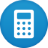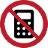# Vector calculation

## Vector Calculation

In the following descriptin are vector operations using vectors of length two or three. Vectors can have any number of entries.

Vectors can only be added if the number of dimensions and their orientation (columns or row-oriented) are the same.The vectors $$\left[\matrix{X_a\\Y_a}\right] + \left[\matrix{X_b\\Y_b}\right]$$     and    $$\left[\matrix{X_a\\Y_a\\Z_a}\right] + \left[\matrix{X_b\\Y_b\\Z_b}\right]$$ can be added.The vectors $$\left[\matrix{X_a\\Y_a}\right] + \left[\matrix{X_b\\Y_b\\Z_b}\right]$$     and    $$[X_a\;Y_a\;Z_a]+ \left[\matrix{X_b\\Y_b\\Z_b}\right]$$ can not be added

Each number in the list is called a element. Vectors can be added and subtracted by adding and subtracting the elements.

$$\left[\matrix{a\\b}\right] + \left[\matrix{c\\d}\right] = \left[\matrix{a+c\\b+d}\right]$$

## Vector Subtraction

The subtraction of vectors is identical to the addition of vectors, but with negative operator. For the vector subtraction, the same rules apply as for the addition of vectors.

## Vector Multiplication

Vectors also can be multiplied by real numbers, as follows.

$$\left[\matrix{a\\b}\right]·\left[\matrix{c\\d}\right]=ac+bd$$

Note, the answer is a real number, not a vector.

$$a·\left[\matrix{x\\y\\z}\right]=\left[\matrix{a· x\\a·y\\a·z}\right]$$
$$5·\left[\matrix{2\\5\\4}\right]=\left[\matrix{5· 2\\5·5\\5·4}\right]=\left[\matrix{10\\25\\20}\right]$$